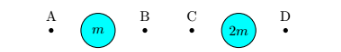# Problem: Consider the six equally-spaced objects shown in the diagram below. The points A, B, C and D represent locations in empty space and the large circles represent planets with masses m and 2m.  1. At which location(s) would the magnitude of the net gravitational force on an object be a maximum? 2. At which location(s) would the magnitude of the net gravitational force on an object be a minimum? Separate your answers by a semicolon. 1. A and D; B and C 2. C; A 3. B and C; A 4. B; D 5. D; B 6. A; C 7. B and C; A and D 8. B; A and D 9. A; B and C 10. D; B and C

###### FREE Expert Solution
94% (452 ratings)
###### Problem Details

Consider the six equally-spaced objects shown in the diagram below. The points A, B, C and D represent locations in empty space and the large circles represent planets with masses m and 2m.

1. At which location(s) would the magnitude of the net gravitational force on an object be a maximum?

2. At which location(s) would the magnitude of the net gravitational force on an object be a minimum?

1. A and D; B and C

2. C; A

3. B and C; A

4. B; D

5. D; B

6. A; C

7. B and C; A and D

8. B; A and D

9. A; B and C

10. D; B and C